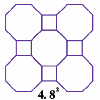#### You may also like### Schlafli Tessellations

are somewhat mundane they do pose a demanding challenge in terms of 'elegant' LOGO procedures. This problem considers the eight semi-regular tessellations which pose a demanding challenge in terms of 'elegant' LOGO procedures.### Napoleon's Theorem

Triangle ABC has equilateral triangles drawn on its edges. Points P, Q and R are the centres of the equilateral triangles. What can you prove about the triangle PQR?

# Polygon Walk

##### Age 16 to 18Challenge Level

After drawing a couple of regular hexagons such that there is a vertex at the top, and joining up those vertices, it became clear that 2 finite lines can reach any vertex without stopping at an internediate location nearer to the centre, and those 2 lines are like the vectors $\mathbf{j} = \begin{pmatrix}0\\1\end{pmatrix}$, and $\mathbf{u} = \begin{pmatrix}\frac{\sqrt{3}}{2}\\\frac{1}{2}\end{pmatrix}$.

But we wanted one of the vectors to be $\mathbf{i}$, so we can rotate the whole problem by 90$^{\circ}$ (swap two components and multiply either by -1) so we have $\mathbf{i} = \begin{pmatrix}1\\0\end{pmatrix}$, and $\mathbf{u} = \begin{pmatrix}-\frac{1}{2}\\\frac{\sqrt{3}}{2}\end{pmatrix}$.

The vertices of the equilateral triangle coincide with vertices of the hexagon, so the vectors are the same for the triangle, so $\mathbf{v} = \mathbf{u}$. But Ulaf must detour, Vicky need not.

For the pentagon, draw one in some orientation, say with a central top vertex. If we say that the vertices sit on a unit circle, we can see that a multiple of the y-coordinate of $\mathbf{w}$ must be -1, and another multiple must be $\cos(36^{\circ})$. Having a look at $\cos(36^{\circ})$ in the calculator, I am pretty sure it is irrational, and thus we cannot have the multiples described.

I first converted 36$^{\circ}$ to radians, to get $\frac{\pi}{5}$ radians. I first looked at using the cosine rule, but then realised that I could use the fact that $\cos(5 \theta) = -1$, expand $\cos(5\theta)$ in terms of $\cos(\theta)$, and solve to get an exact answer which I could show was irrational. However this expends to a quintic which is hard to solve. So I decided to try solving for $\sin(5\theta) = 0$, then use the identity to convert the answer to $\cos(\theta)$. Expanding $\sin(5\theta) = 0$ in terms of $\sin(\theta)$, using identities, we get $16s^4 - 20s^2 + 5 = 0 \therefore s^2 = \frac{5\pm \sqrt{5}}{8}$, checking with the calculator, the one we want is $s = \sqrt{\frac{5- \sqrt{5}}{8}}$, then we find that $\cos(36^{\circ}) = \sqrt{\frac{3+\sqrt{5}}{8}}$, and since all square roots of numbers that are not themselves squares are irrational, $\cos (36^{\circ})$ is irrational. Thus Wilber was mistaken.